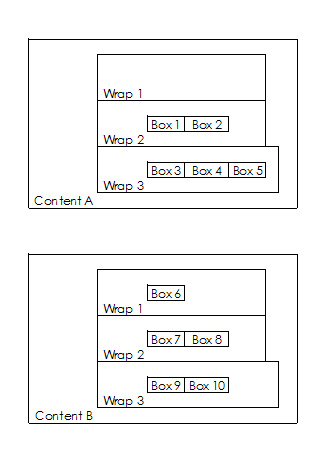## Support### Account

Home Forums General Issues Query posts by value (array?) and not by fieldname

Solved

# Query posts by value (array?) and not by fieldname

•Hi guys

I used ACF some years ago for some hobby pages. Now I started a new Project and got some Problems with my Idea. Probably it’s more a Problem of my Brain than a real issue. 🙂

Mainquestion is how to query posts by value (array?) and not by fieldname. Is it and how is it possible?

The posts have some Customfields (Dropdown). One field is “Content” with possible values “A”, “B”, “C”, “D”. Another field is “Wrap” with values “1”, “2”, “3”, “4”, “5”.

I like to query the posts like this:This would work so far (symbolic code):

``````
\$posts = get_posts(array('meta_key' => 'Content', 'meta_value' => 'A'));
foreach( \$posts as \$post ) {
\$posts = get_posts(array('meta_key' => 'Wrap', 'meta_value' => '1'));
foreach( \$posts as \$post ) {
the_field();
}
\$posts = get_posts(array('meta_key' => 'Wrap', 'meta_value' => '2'));
foreach( \$posts as \$post ) {
the_field();
}
\$posts = get_posts(array('meta_key' => 'Wrap', 'meta_value' => '3'));
foreach( \$posts as \$post ) {
the_field();
}
\$posts = get_posts(array('meta_key' => 'Wrap', 'meta_value' => '4'));
foreach( \$posts as \$post ) {
the_field();
}
\$posts = get_posts(array('meta_key' => 'Wrap', 'meta_value' => '5'));
foreach( \$posts as \$post ) {
the_field();
}
};

};
``````

Same vor Content B and so on.

But i don’t like to formate each post separate. I would like three loops. One for the Contents, one für the Wraps and one for the Posts (which loop is formated once). May I get the values as an array in the loop. But i don’t know how. Maybe someone of you can help me?

Thank you very much. 🙂

Regards Light

•One step further…. this works for the first loop:

``````
<?php
\$posts = get_posts(array('meta_key'	=> 'Content'));
if( \$posts ) {
\$anzContent = array();
foreach( \$posts as \$post ) {
setup_postdata( \$post );
\$anzContent[] = get_field('Content');
}; //foreach( \$posts as \$post )
\$uniqueContent = array_unique(\$anzContent);
foreach( \$uniqueContent as \$contentboxes ) {
setup_postdata( \$contentboxes );
echo('<div class="Contentbox">');
echo(\$contentboxes);
echo('</div>'); //Contentbox
} //foreach( \$uniqueContent as \$contentboxes )
}; //if( \$posts )
wp_reset_query();
?>
``````

To get the Contentboxes i did 2 loops. One loop to get all Content values of all posts (like A,A,B,E,D,D,D).
then I put this array in an array_unique to get each value only once (A,B,E,D)
For each of this array_unique_values i createt a <div>.
This works so far.

Now i tried to get the wraps of each Contentbox the same way:

``````
<?php
\$posts = get_posts(array('meta_key'	=> 'Content'));
if( \$posts ) {
\$anzContent = array();
foreach( \$posts as \$post ) {
setup_postdata( \$post );
\$anzContent[] = get_field('Content');
}; //foreach( \$posts as \$post )
\$uniqueContent = array_unique(\$anzContent);
foreach( \$uniqueContent as \$contentboxes ) {
setup_postdata( \$contentboxes );
echo('<div class="Contentbox">');

\$wraps = get_posts(array('meta_value' => \$contentboxes));
if( \$wraps ) {
\$anzwraps = array();
foreach( \$wraps as \$wrap ) {
setup_postdata( \$wrap );
\$anzwraps[] = get_field('wrap');
}; //foreach( \$wraps as \$wrap )
\$uniquewrap = array_unique(\$anzwraps);
foreach( \$uniquewrap as \$thiswrap ) {
setup_postdata( \$thiswrap );
echo('<div>');
echo(\$thiswrap);
echo('</div>');
};
};

echo('</div>');
};
};
wp_reset_query();
?>
``````

This is not working. It echoes the same value each contentbox. Why? I don’t get it… 🙁

•I solved it. Maybe it could be a better code. But it works and i’m happy with it. 🙂

``````<?php
\$posts = get_posts(array('meta_key'	=> 'haus'));
if( \$posts ) {
\$anzhaus = array();
foreach( \$posts as \$post ) {
setup_postdata( \$post );
\$anzhaus[] = get_field('haus');
}; //foreach( \$posts as \$post )
\$uniquehaus = array_unique(\$anzhaus);
foreach( \$uniquehaus as \$hpost ) {
setup_postdata( \$hpost );
echo('<div class="hausbox"><div class="Haustitel">'.\$hpost.'</div>');
\$args = array('meta_query'	=> array('relation'		=> 'AND',	array('key'		=> 'geschoss'),	array('value'		=> \$hpost)	));
\$the_query = new WP_Query( \$args );
if( \$the_query->have_posts() ) {
\$anzgeschoss = array();
while ( \$the_query->have_posts() ) {
\$the_query->the_post();
\$anzgeschoss[] = get_field('geschoss');
};
\$uniquegeschoss = array_unique(\$anzgeschoss);
foreach (\$uniquegeschoss as \$geschoss) {
echo'<div class="geschossbox"><div class="Geschosstitel">'.\$geschoss.'</div>';
\$wohnargs = array('meta_query'	=> array('relation'		=> 'AND',	array('value'		=> \$geschoss),	array('value'		=> \$hpost)	));
\$wohn_query = new WP_Query( \$wohnargs );
if( \$wohn_query->have_posts() ) {
while( \$wohn_query->have_posts() ) {
\$wohn_query->the_post(); ?>
<div class="Wohnungsbox"> //here we go!!!

</div>
<?php };
}; ?>
</div>
<?php		};
};
wp_reset_query();	 // Restore global post data stomped by the_post().
echo('</div>'); //hausbox
} //foreach( \$uniquehaus as \$hpost )
}; //if( \$posts )
wp_reset_query();	 // Restore global post data stomped by the_post().
?>``````
Viewing 3 posts - 1 through 3 (of 3 total)

You must be logged in to reply to this topic.